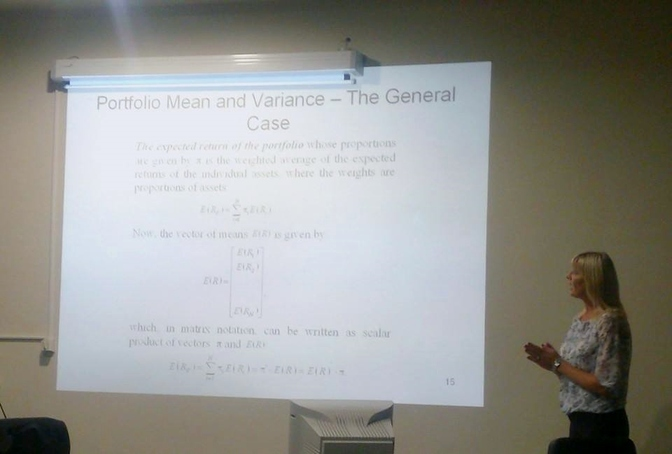# Introduction to financial modeling, Zdravka Aljinović

Prof. Zdravka Aljinović from University of Split gave us a lecture (30/9/2014) about basic portofolio optimization and Markowitz methods.

## Introduction to financial modeling

• Basics of the Modern Portfolio Theory; Portfolio Mean and Variance, Calculating the Variance - Covariance Matrix.
• Theoretical framework of the Markowitz’ model, the minimum variance set, efficient portfolio, efficient frontier - short sale allowed, efficient frontier without short sale.
• Is Variance a proper risk measure? - An Alternative Risk Measures.
• CAPM, beta, testing CAPM.
• Demonstration and Calculation in Excel.Please find attached the material of the lecture.